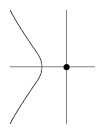# AcnodeFor instance, the point $(0,0)$ is an acnode of the curve $X^3+X^2+Y^2=0$ in $\mathbf R^2$.